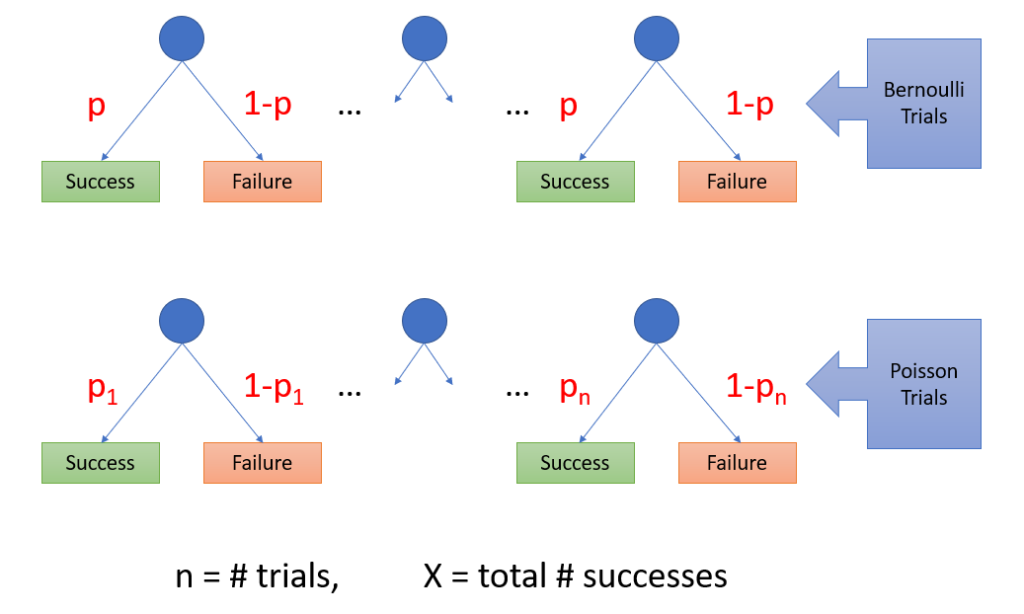# Poisson Trial: Definition, Examples

Share on

## What is a Poisson Trial?

A Poisson trial is a collection of Bernoulli trials with unequal probabilities. In other words, one Poisson trial is exactly the same as one Bernoulli trial. However, when you have many of them with different probabilities, they are called Poisson trials .A binomial trial is an experiment with two outcomes, success or failure. A “success” is the result you’re looking for. For example, it could be a “yes” on a questionnaire, a perfect widget from a factory floor, or a plant that flowers (as opposed to one that doesn’t). In real life, it’s common for these success/failure situations to have unequal probabilities. For example, different lines on a factory floor may have small probability differences in the number of faulty widgets each line produces. Analysis of road accidents often involves Poisson trials, as probabilities change with each instance of travel . When these probabilities are unequal for each Bernoulli trial of independent events, it becomes a Poisson trial.

Poisson trials follow a Poisson distribution and have no limit on the number of outcomes. Bernoulli trials follow a Binomial distribution and are limited by a fixed number of trials. the two distributions are extremely similar; so similar, in fact, that the Poisson distribution can be derived as an approximation to the binomial when the number of trials is very large and probabilities are very small .

## References

 CS174 Spring 99 Lecture 9 Summary. Retrieved December 9, 2021 from: https://people.eecs.berkeley.edu/~jfc/cs174lecs/lec9/lec9.html
 Kassawat, S. et al. (2015). Integration of Spatial Models for Web-based Risk Assessment of Road Accident.
 Barrick, D. (2017). Biomolecular Thermodynamics: From Theory to Application. CRC Press.

CITE THIS AS:
Stephanie Glen. "Poisson Trial: Definition, Examples" From StatisticsHowTo.com: Elementary Statistics for the rest of us! https://www.statisticshowto.com/poisson-trial/
---------------------------------------------------------------------------Need help with a homework or test question? With Chegg Study, you can get step-by-step solutions to your questions from an expert in the field. Your first 30 minutes with a Chegg tutor is free!